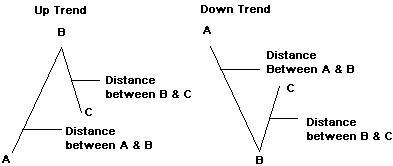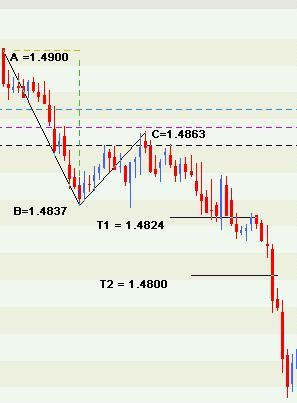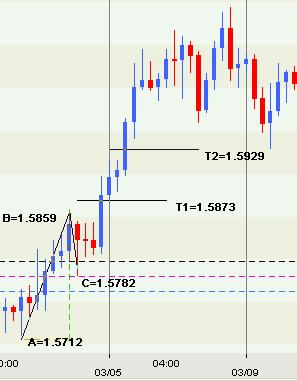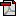Home | Previous | Next Lesson

This Lesson Can Be Printed See Instructions Below

# Fibonacci - Maximum Profit Targets

This lesson we are going to work out the best way to take maximum profits from a position long or short. Many traders know how to get into a position but are often uncertain when they should get out.

 In our last lesson we discussed Fibonacci levels and how to use these levels for entry and stop placement. So we have a sensible place to enter the market and a sensible place to put our stop loss now we need a logical target. Don't worry if this sounds a little complicated it isn't. From the last lesson you may remember that we calculated out retracement levels by measuring the distance between point A and point B. All we are going to do now is add another measurement called point C to get some fibonacci expansion ratiosWe will eventually finish up with two targets. T1 and T2. We shall discuss which one to choose later. The formula for the calculations would look like this.
T1 = .618(B-A)+C
T2 = B-A+C

Let's assume an imaginary security called ABC.PTY. Point A shall be 189, Point B shall be 278 and point C shall be 245. It's in an up trend and the first move (A to B) is 89 (245-189). It then pulls back to point C. The distance between point B and point C is 33 (278-245). We can now calculate some numbers.
T1 = .618(278-189)+245 Target is 300
T2 = 278-189+245 Target is 334

The chart below is a 5-minute chart of the US dollar/ Canadian dollar (USD/CAD) as you can see A is 1.4900, B is 1.4837 and C is 1.4863. Therefore:
T1 = .618(1.4837-1.4900)+1.4863 Target is 1.4824
T2 = 1.4837-1.4900+1.4863 Target is 1.4800In our next chart we have the 4 hour chart of the British Pound/ US dollar (GBP/USD) as you can see A is 1.5712, B is 1.5859 and C is 1.5782. Therefore:
T1 = .618(1.5859-1.5712)+1.5782 Target is 1.5873
T2 = 1.5859-1.5712+1.5782 Target is 1.5929As I mentioned earlier there are other expansion ratios but these are the two I like to use. I find it very useful is to keep an eye on the first target (T1). If you are in an up trend and T1 is below resistance then I would use that as a target. If T1 is above resistance then I would probably use T2. The reverse is true for a down trend.

Good Trading

Best Regards
Mark McRae

Information, charts or examples contained in this lesson are for illustration and educational purposes only. It should not be considered as advice or a recommendation to buy or sell any security or financial instrument. We do not and cannot offer investment advice. For further information please read our disclaimer.To PRINT or save a copy of this lesson in PDF format simply click the PRINT link. This will open the lesson in a PDF format which, you can then PRINT. If you are unfamiliar with PDF or don't have a FREE copy of Arobat Reader see instructions.